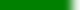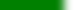The following is simply a conveniently formatted list of learning goals. More likely than not, some might be hard to assess through a quiz or exam. Students should prioritize their studying based on:

• how much time in lecture was spent discussing or understanding the topic,
• how much the topic was stressed in learning group assignments
• and the student's comfort level with the topic

# Assessable Learning Goals covered for Quiz 2Know Fisher-Yates shuffling algorithm, be able to apply it to sampling tasks in simulations.Know the reservoir sampling algorithm for selecting n random elements from an unkown sized population.Know the several different properties and attributes of sampling and shuffling algorithms presented in the text.Know how to use accept/reject techniques for uniformly random (geometric) point generation.Know how to write Monte Carlo simulations for estimating a discrete data histogram of many events.Know how to write Monte Carlo simulations for estimating the Pr(A) of an event A.Know the pitfalls associated with common (naive) methods of random point generation.Know the tell-tale feature(s) of spatial plots produced by faulty point generation algorithms.Describe S, the system state. What does "system" refer to?How is the simulation clock different than the wall clock or an accelerated, virtual clock?Understand the basic steps in augmenting a pre-existing NES with additional system and meta events.What constraint(s) are placed on events being inserted into the event list?What is the difference between system events and meta events?Cite pros and cons for at least two "pure" event list data structures commonly used for event list management.Cite pros and cons for at least two hybrid data structures commonly used for event list management.Understand that skewed results are obtained from SIS simulations (w/ delivery delays) with syncronized demand and delay random sequences.What is a distribution's `idf()`?What is special about outliers in the context of computer simulation results?Know the general form of the integral equations for CRVs (average and deviation calculations).Know what constitutes a histogram and how it is connected to CDFs.How is the height of a continuous histogram bin calculated?Know the general approach to binning continuous data, about how many bins are needed for a sample of `n`?How is the approach of MSOD regression lines different than the approach taken for conventional "least squares" regression lines?What is a mean-square orthogonal-distance (MSOD) linear regression line?Given Welford's discrete and integral mean and variance equations (Thms 4.1.2, 4.1.4), be able to apply them to a set of data.Know that Welford's Equations exist, why they are superior to the "one-pass" algorithm common in statistical texts.Know which of the two (non Welford) standard equations for calculating s2 or s is flawed.DES people don't need integrals and anti-differentiation when integrating. Why not?Know that uniform arrival times and uniform interarrivals are not the same thing.Know the inversion technique for non-stationary arrival times.Know the relationships between PMF/PDF and CDF for both discrete and continuous distributions.Know the relationships between PMF/PDF, CDF, and InvDF. How do you derive one from another? Can this always be done?Know how to maniuplate and derive CDFs and PDFs of variables that are functions of a Uniform(a,b) input.Know the CDF search technique for creating discrete random variates.Know the Exponential(mu) random variate and the interpretation of its parameter mu in the context of arrival times.Know the F(x) inversion technique for constructing random variates. What is the requirement on F(x)?Know the three techniques for constructing a discrete random variate.Know what the construction technique means for random variates (of which summation is one example).What does the parameter u in random variates represent? Computationally, how do we get a value for u in code?Know the criteria by which variates are judged: portability, efficiency, clarity, syncronicity, ...Know the two techniques for truncating pre-existing random variates.